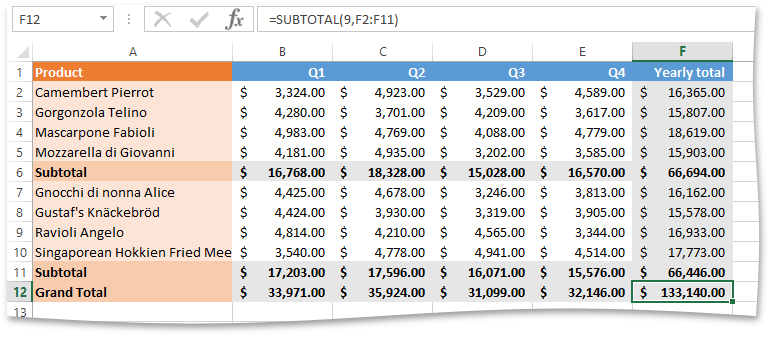.NET Framework 4.5.2+

# Use the Excel Export API to Create SubtotalsTo create a subtotal, call the IXlCell.SetFormula method to add a formula that contains a SUBTOTAL function. To create a formula, use the XlFunc.Subtotal method specifying a summary function as one of the XlSummary enumeration values.

The code snippet below creates SUM subtotals which include values in the hidden rows.

``````// Create the grand total row.
using (IXlRow row = sheet.CreateRow()) {
using (IXlCell cell = row.CreateCell()) {
cell.Value = "Grand Total";
cell.ApplyFormatting(totalRowFormatting);
cell.Formatting.Fill = XlFill.SolidFill(XlColor.FromTheme(XlThemeColor.Accent2, 0.6));
}
for (int j = 0; j < 5; j++) {
using (IXlCell cell = row.CreateCell()) {
// Use the SUBTOTAL function to calculate grand total sales for each quarter and the entire year.
cell.SetFormula(XlFunc.Subtotal(XlCellRange.FromLTRB(j + 1, startDataRowForGrandTotal, j + 1, row.RowIndex - 1), XlSummary.Sum, false));
cell.ApplyFormatting(totalRowFormatting);
}
}
}
``````